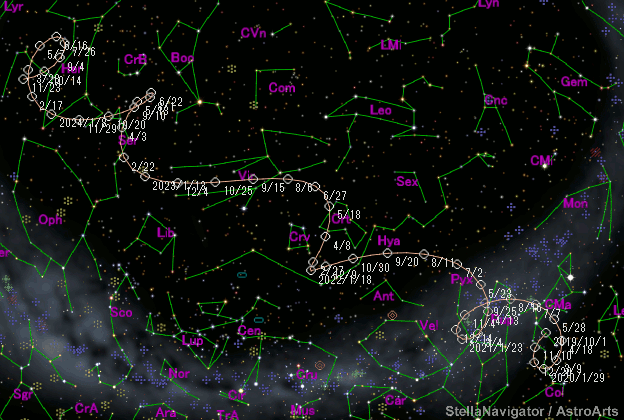# \$B%"%H%i%9WB@1(B

C/2019 T4 ( ATLAS )###\$B%W%m%U%#!<%k(B

 \$BH/8+F|(B 2019\$BG/(B10\$B7n(B9\$BF|(B \$BH/8+8wEY(B 19.3\$BEy(B \$BH/8+ Asteroid Terrestrial-Impact Last Alert System (ATLAS) search program

###\$B###\$B50F;MWAG(B

```Epoch 2022 May 21.0 TT = JDT 2459720.5
T 2022 June  9.24030 TT                                 Williams
q   4.2428794            (2000.0)            P               Q
z  +0.0010249      Peri.  351.19928     -0.95992430     +0.05603361
+/-0.0000112      Node   199.94012     -0.18195605     -0.86982944
e   0.9956515      Incl.   53.62874     -0.21316033     +0.49016017
From 35 observations 2019 Feb. 5-Dec. 28, mean residual 0".3.
```

###\$B@1?^(B###\$B8wEYJQ2=(B

```        m1 = -9.0 + 5 log\$B&\$(B + 25   log r  [    ,-975]  (              \$B!A(B2019\$BG/(B10\$B7n(B 8\$BF|(B)
m1 =  5.0 + 5 log\$B&\$(B + 10.0 log r  [-975,    ]  (2019\$BG/(B10\$B7n(B 8\$BF|!A(B              )
```##### \$B50F;MWAG\$O(BM.P.E.C. 2020-A105\$B\$K7G:\\$5\$l\$?\$b\$N\$G\$9!#(B \$B@1?^\$O%9%F%i%J%S%2!<%?(B Ver.10 (\$B%"%9%H%m%"!<%D(B) \$B\$G:n@.\$7\$?\$b\$N\$G\$9!#(B \$B8wEY%0%i%U\$O(BComet for Windows\$B\$G:n@.\$7\$?\$b\$N\$G\$9!#(B